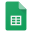# Arithmetic of hyperelliptic curves

Special Session: Arithmetic of Hyperelliptic Curves

Michigan State University,

East Lansing, MI

March 13-15, 2015

Meeting #1108

Overview

Arithmetic geometry is the study of the solutions in k^n of a system of polynomials in n variables with coefficients in a ring k such that k=Z, Q, Z/pZ, or a Dedekind domain. The subject is a combination of algebraic number theory, commutative algebra, and algebraic geometry. During the last 30-40 years the subject has seen many developments both theoretical and computational.

The goal of this session is to look at some of these problems focused on hyperelliptic and superelliptic curves. Computational aspects of arithmetic geometry and applications in cryptography and coding theory will be encouraged. The session will be a continuation of the NATO Advanced Study Institute on the Arithmetic of Hyperelliptic Curves held in August 2014 organized by this proposer.

Topics of the session include, but are not limited to:

• Integral extensions and integral closure
• Algebraic curves
• Jacobians of algebraic curves, rational torsion points in the Jacobian etc.
• Computational number theory, rational points on curves,
• Curves defined over Q
• Minimal discriminants and conductors
• Selmer groups in Jacobians
• Arithmetic invariant theory
• The arithmetic of hyperelliptic curves
• Pairings and Weil descent
• Mordell-Weil group
• Arithmetic Mirror Symmetry
• others

Speakers

• Balakrishnan, Jennifer S.; Oxford University
• Beshaj, Lubjana; Oakland University
• Broughton, Allen; Rose-Hulman Institute of Technology
• Izquierdo, Milagros, Linköping University
• Levin, Aaron, Michigan State University
• Shaska, Tony, Oakland University
• Shor, Caleb, Western New England University
• Daniels, Harris, Amherst College

Contact: T. Shaska (shaska AT oakland DOT edu)

Proceedings: There will be a proceedings volume for this session either from AMS Contemporary Math. series or a special issue of some of the journal in the area.

Website:

Talksams-1108-michigan state.schedule